# RS Aggarwal Solutions for Class 8 Chapter 13 - Time and Work Exercise 13B

RS Aggarwal Solutions for Class 8 Maths Chapter 13- Exercise 13B, Time and Work links are provided below, students can refer and easily download them. Our experts have solved the RS Aggarwal Solutions to ensure that the students are thorough with their basic concepts by practicing the solutions. In Exercise 13B of RS Aggarwal Class 8 Maths, we shall brief all the topics covered in Chapter 13.

## Download PDF of RS Aggarwal Solutions for Class 8 Maths Chapter 13- Exercise 13B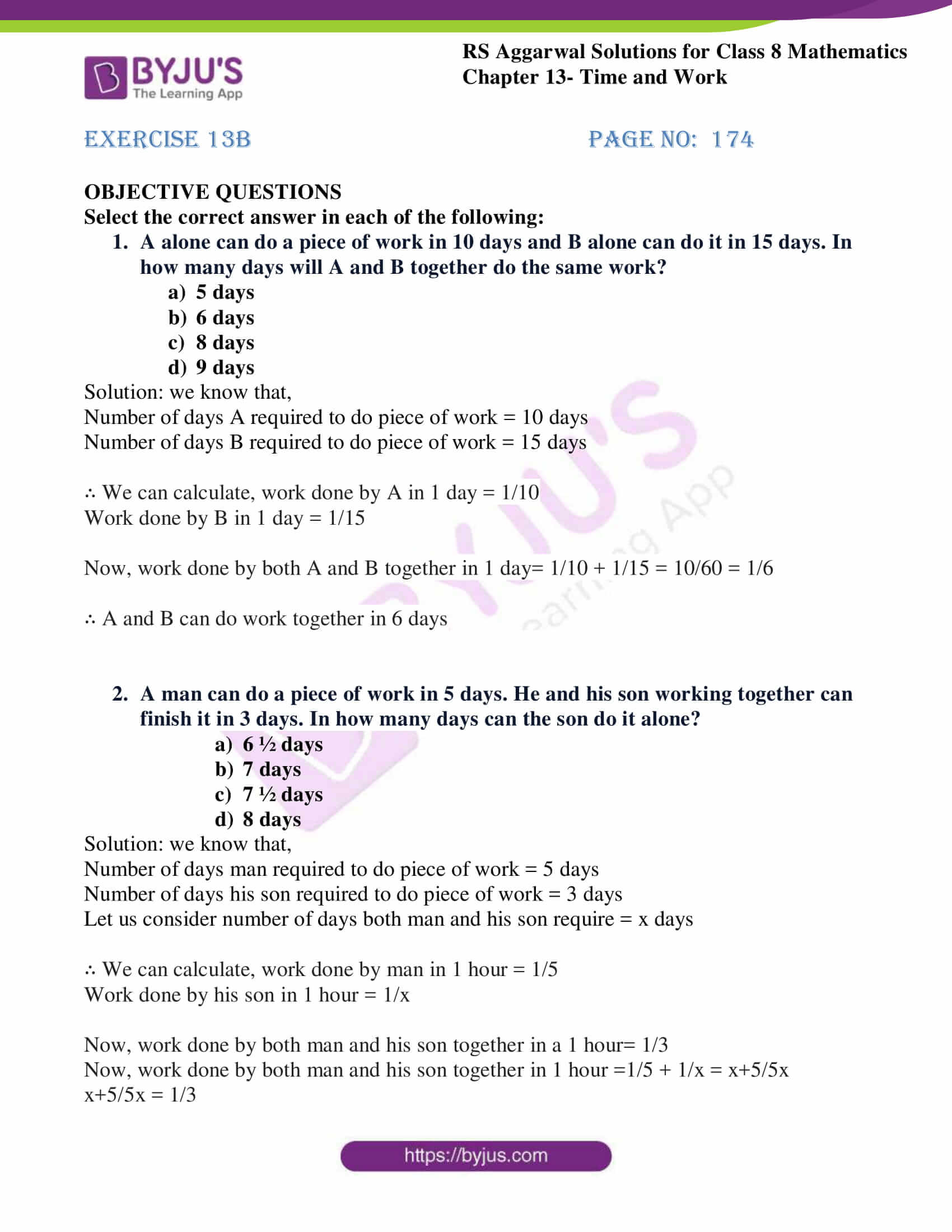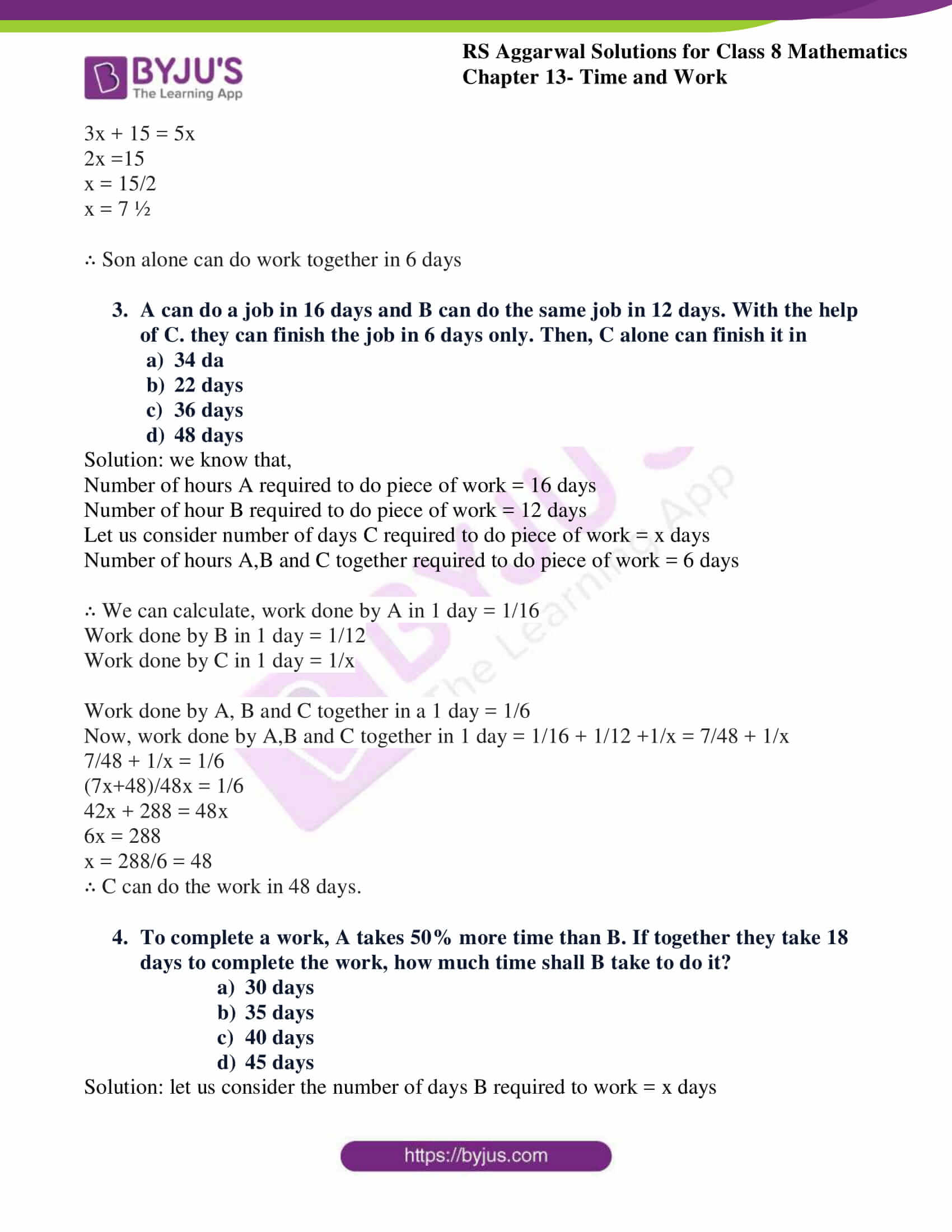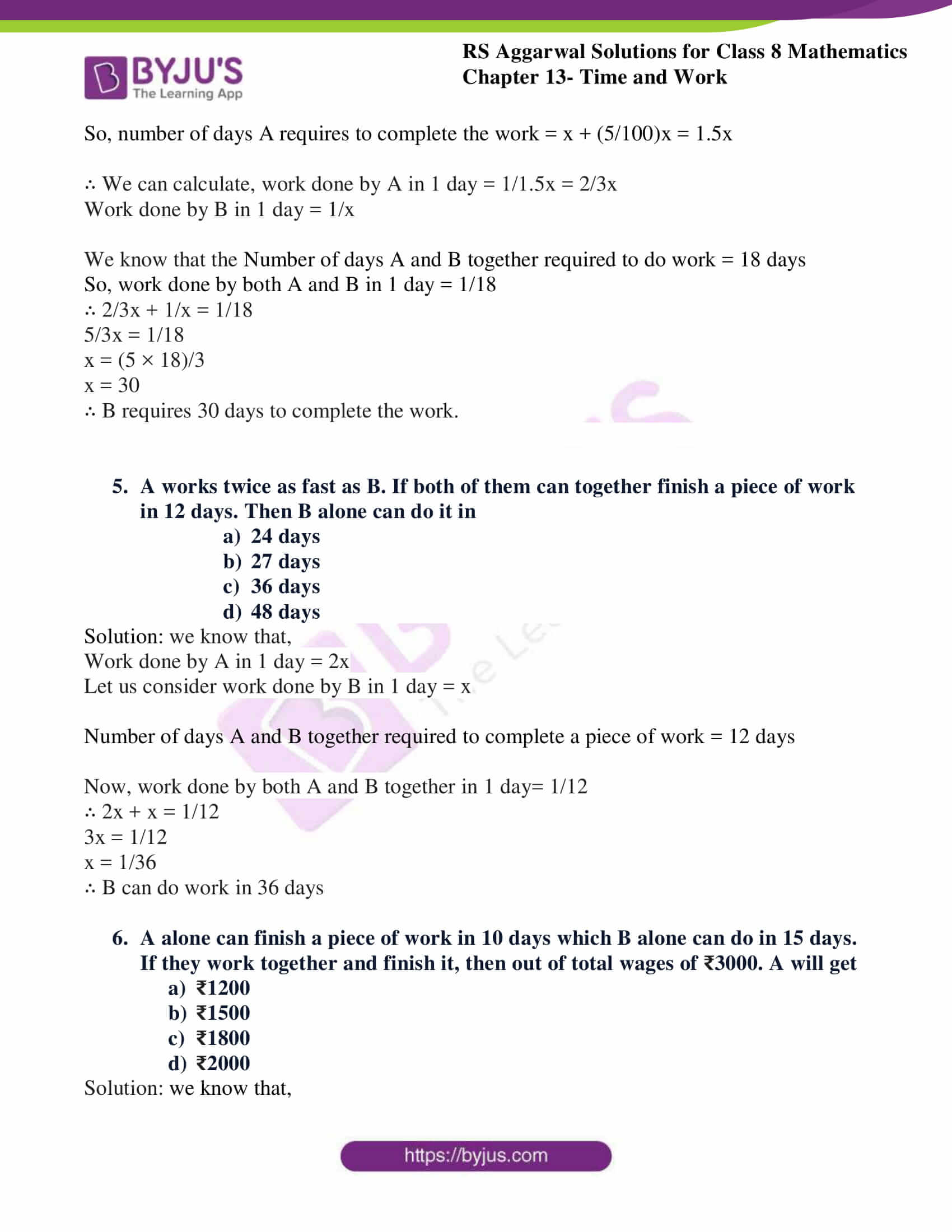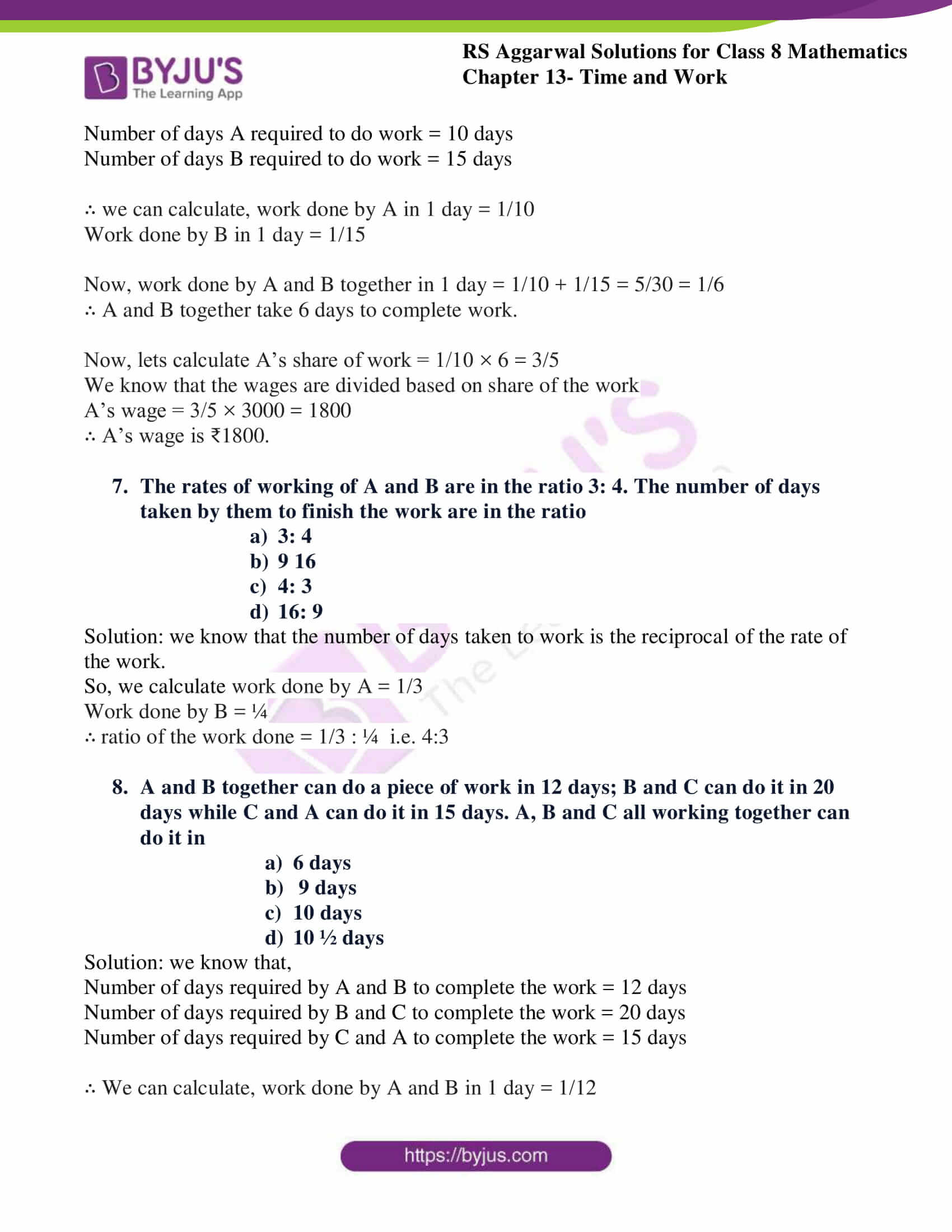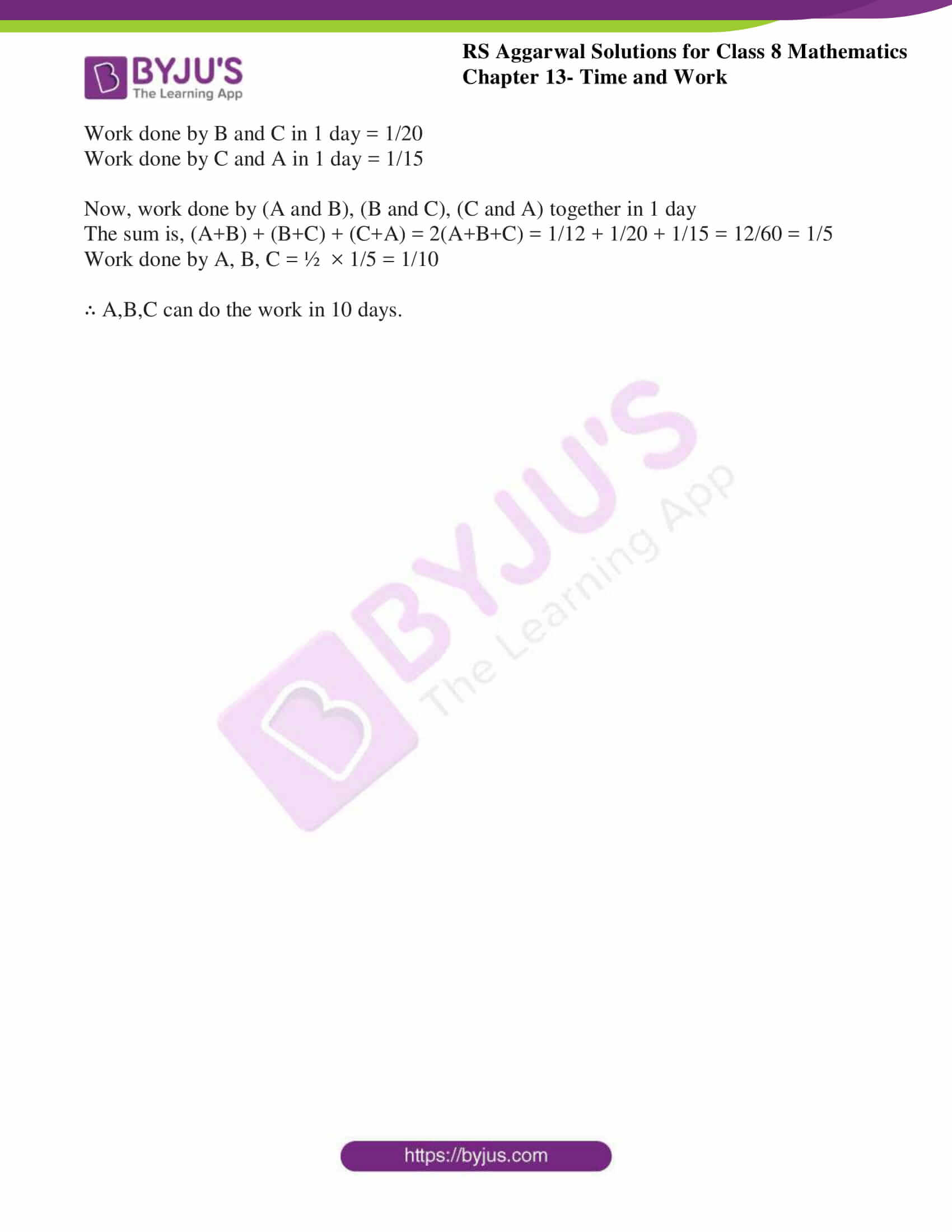### Access Answers to RS Aggarwal Solutions for Class 8 Maths Chapter 13- Time and Work Exercise 13B

Select the correct answer in each of the following:

1. A alone can do a piece of work in 10 days and B alone can do it in 15 days. In how many days will A and B together do the same work?

1. 5 days
2. 6 days
3. 8 days
4. 9 days

Solution: we know that,

Number of days A required to do piece of work = 10 days

Number of days B required to do piece of work = 15 days

∴ We can calculate, work done by A in 1 day = 1/10

Work done by B in 1 day = 1/15

Now, work done by both A and B together in 1 day= 1/10 + 1/15 = 10/60 = 1/6

∴ A and B can do work together in 6 days

2. A man can do a piece of work in 5 days. He and his son working together can finish it in 3 days. In how many days can the son do it alone?

1. 6 ½ days
2. 7 days
3. 7 ½ days
4. 8 days

Solution: we know that,

Number of days man required to do piece of work = 5 days

Number of days his son required to do piece of work = 3 days

Let us consider number of days both man and his son require = x days

∴ We can calculate, work done by man in 1 hour = 1/5

Work done by his son in 1 hour = 1/x

Now, work done by both man and his son together in a 1 hour= 1/3

Now, work done by both man and his son together in 1 hour =1/5 + 1/x = x+5/5x

x+5/5x = 1/3

3x + 15 = 5x

2x =15

x = 15/2

x = 7 ½

∴ Son alone can do work together in 6 days

3. A can do a job in 16 days and B can do the same job in 12 days. With the help of C. they can finish the job in 6 days only. Then, C alone can finish it in

1. 34 days
2. 22 days
3. 36 days
4. 48 days

Solution: we know that,

Number of hours A required to do piece of work = 16 days

Number of hour B required to do piece of work = 12 days

Let us consider number of days C required to do piece of work = x days

Number of hours A,B and C together required to do piece of work = 6 days

∴ We can calculate, work done by A in 1 day = 1/16

Work done by B in 1 day = 1/12

Work done by C in 1 day = 1/x

Work done by A, B and C together in a 1 day = 1/6

Now, work done by A,B and C together in 1 day = 1/16 + 1/12 +1/x = 7/48 + 1/x

7/48 + 1/x = 1/6

(7x+48)/48x = 1/6

42x + 288 = 48x

6x = 288

x = 288/6 = 48

∴ C can do the work in 48 days.

4. To complete a work, A takes 50% more time than B. If together they take 18 days to complete the work, how much time shall B take to do it?

1. 30 days
2. 35 days
3. 40 days
4. 45 days

Solution: let us consider the number of days B required to work = x days

So, number of days A requires to complete the work = x + (5/100)x = 1.5x

∴ We can calculate, work done by A in 1 day = 1/1.5x = 2/3x

Work done by B in 1 day = 1/x

We know that the Number of days A and B together required to do work = 18 days

So, work done by both A and B in 1 day = 1/18

∴ 2/3x + 1/x = 1/18

5/3x = 1/18

x = (5 × 18)/3

x = 30

∴ B requires 30 days to complete the work.

5. A works twice as fast as B. If both of them can together finish a piece of work in 12 days. Then B alone can do it in

1. 24 days
2. 27 days
3. 36 days
4. 48 days

Solution: we know that,

Work done by A in 1 day = 2x

Let us consider work done by B in 1 day = x

Number of days A and B together required to complete a piece of work = 12 days

Now, work done by both A and B together in 1 day= 1/12

∴ 2x + x = 1/12

3x = 1/12

x = 1/36

∴ B can do work in 36 days

6. A alone can finish a piece of work in 10 days which B alone can do in 15 days. If they work together and finish it, then out of total wages of ₹3000. A will get

1. ₹1200
2. ₹1500
3. ₹1800
4. ₹2000

Solution: we know that,

Number of days A required to do work = 10 days

Number of days B required to do work = 15 days

∴ we can calculate, work done by A in 1 day = 1/10

Work done by B in 1 day = 1/15

Now, work done by A and B together in 1 day = 1/10 + 1/15 = 5/30 = 1/6

∴ A and B together take 6 days to complete work.

Now, lets calculate A’s share of work = 1/10 × 6 = 3/5

We know that the wages are divided based on share of the work

A’s wage = 3/5 × 3000 = 1800

∴ A’s wage is ₹1800.

7. The rates of working of A and B are in the ratio 3: 4. The number of days taken by them to finish the work are in the ratio

1. 3:4
2. 9:16
3. 4:3
4. 16: 9

Solution: we know that the number of days taken to work is the reciprocal of the rate of the work.

So, we calculate work done by A = 1/3

Work done by B = ¼

∴ ratio of the work done = 1/3 : ¼ i.e. 4:3

8. A and B together can do a piece of work in 12 days; B and C can do it in 20 days while C and A can do it in 15 days. A, B and C all working together can do it in

1. 6 days
2. 9 days
3. 10 days
4. 10 ½ days

Solution: we know that,

Number of days required by A and B to complete the work = 12 days

Number of days required by B and C to complete the work = 20 days

Number of days required by C and A to complete the work = 15 days

∴ We can calculate, work done by A and B in 1 day = 1/12

Work done by B and C in 1 day = 1/20

Work done by C and A in 1 day = 1/15

Now, work done by (A and B), (B and C), (C and A) together in 1 day

The sum is, (A+B) + (B+C) + (C+A) = 2(A+B+C) = 1/12 + 1/20 + 1/15 = 12/60 = 1/5

Work done by A, B, C = ½ × 1/5 = 1/10

∴ A,B,C can do the work in 10 days.

## RS Aggarwal Solutions for Class 8 Maths Chapter 13- Exercise 13B

Exercise 13B of RS Aggarwal Class 8, Time and Work. This exercise mainly consists of Objective Questions related to the topics discussed earlier in Chapter 13.

Students are suggested to try solving the questions from RS Aggarwal book of Class 8 and then refer to these solutions to know the best way of approaching the different questions. By referring to these solutions, students develop problem-solving abilities and can tackle any type of questions easily. Thus, practicing as many times as possible helps in building time management skills and also boosts the confidence level to achieve high marks.